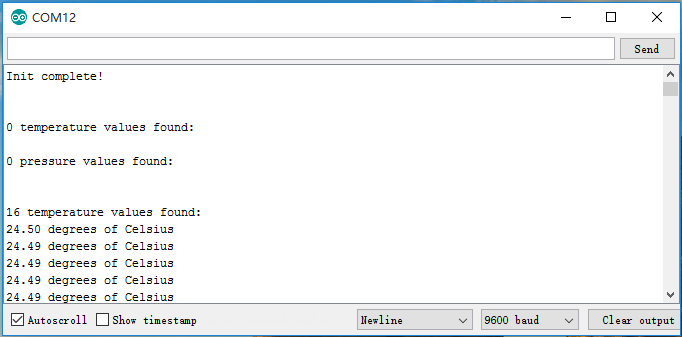edit

# SPI Communication on Seeed Studio XIAO SAMD21

### Hardware¶

Materials required

Hardware Connection

• Step 1. Grove-High Precision pressure Sensor's CSK is connected to Seeed Studio XIAO 's SCK.

• Step 2. Sensor's CS is connected to Seeed Studio XIAO 's D3.

• Step 3. Sensor's SDO is connected to Seeed Studio XIAO 's MISO.

• Step 4. Sensor's SDI is connected to Seeed Studio XIAO 's MOSI.

• Step 5. Sensor's GND is connected to Seeed Studio XIAO 's GND.

• Step 6. Sensor's 3V3 is connected to Seeed Studio XIAO 's 3.3V.

• Step 7. Connect Seeed Studio XIAO to PC via a Type-C cable.

### Software¶

Attention

If this is the first time you work with Arduino, we strongly recommend you to see Getting Started with Arduino before the start.

• Step 2. Refer to How to install library to install library for Arduino.

• Step 3. Copy the code into Arduino IDE and upload.

#include <Dps310.h>

// Dps310 Opject
Dps310 Dps310PressureSensor = Dps310();

void setup()
{
//pin number of your slave select line
//XMC2GO
int16_t pin_cs = 3;
//for XMC 1100 Bootkit  & XMC4700 Relax Kit uncomment the following line
//int16_t pin_cs = 10;

Serial.begin(9600);
while (!Serial);

//Call begin to initialize Dps310
//The parameter pin_nr is the number of the CS pin on your Microcontroller
Dps310PressureSensor.begin(SPI, pin_cs);

//temperature measure rate (value from 0 to 7)
//2^temp_mr temperature measurement results per second
int16_t temp_mr = 2;
//temperature oversampling rate (value from 0 to 7)
//2^temp_osr internal temperature measurements per result
//A higher value increases precision
int16_t temp_osr = 2;
//pressure measure rate (value from 0 to 7)
//2^prs_mr pressure measurement results per second
int16_t prs_mr = 2;
//pressure oversampling rate (value from 0 to 7)
//2^prs_osr internal pressure measurements per result
//A higher value increases precision
int16_t prs_osr = 2;
//startMeasureBothCont enables background mode
//temperature and pressure ar measured automatically
//High precision and hgh measure rates at the same time are not available.
int16_t ret = Dps310PressureSensor.startMeasureBothCont(temp_mr, temp_osr, prs_mr, prs_osr);
//Use one of the commented lines below instead to measure only temperature or pressure
//int16_t ret = Dps310PressureSensor.startMeasureTempCont(temp_mr, temp_osr);
//int16_t ret = Dps310PressureSensor.startMeasurePressureCont(prs_mr, prs_osr);

if (ret != 0)
{
Serial.print("Init FAILED! ret = ");
Serial.println(ret);
}
else
{
Serial.println("Init complete!");
}
}

void loop()
{
uint8_t pressureCount = 20;
float pressure[pressureCount];
uint8_t temperatureCount = 20;
float temperature[temperatureCount];

//This function writes the results of continuous measurements to the arrays given as parameters
//The parameters temperatureCount and pressureCount should hold the sizes of the arrays temperature and pressure when the function is called
//After the end of the function, temperatureCount and pressureCount hold the numbers of values written to the arrays
//Note: The Dps310 cannot save more than 32 results. When its result buffer is full, it won't save any new measurement results
int16_t ret = Dps310PressureSensor.getContResults(temperature, temperatureCount, pressure, pressureCount);

if (ret != 0)
{
Serial.println();
Serial.println();
Serial.print("FAIL! ret = ");
Serial.println(ret);
}
else
{
Serial.println();
Serial.println();
Serial.print(temperatureCount);
Serial.println(" temperature values found: ");
for (int16_t i = 0; i < temperatureCount; i++)
{
Serial.print(temperature[i]);
Serial.println(" degrees of Celsius");
}

Serial.println();
Serial.print(pressureCount);
Serial.println(" pressure values found: ");
for (int16_t i = 0; i < pressureCount; i++)
{
Serial.print(pressure[i]);
Serial.println(" Pascal");
}
}

//Wait some time, so that the Dps310 can refill its buffer
delay(10000);
}


• Step 4. Click Tools > Serial Monitor , or press Ctrl+Shift+M together, open the serial monitor after uploading successfully, the output appears as follows: